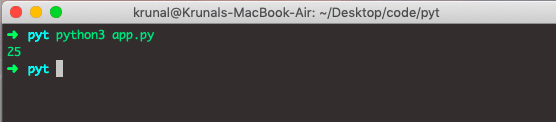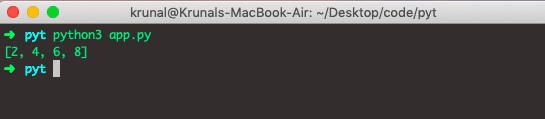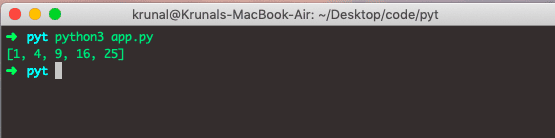# Python Lambda Function: The Complete Guide

In this example, we will see how to use the Lambda function in Python. You will learn what it is, its syntax, and how to use it.

## Python Lambda Function

Python anonymous or lambda function is the function that is defined without a name. While the standard functions are defined using a def in Python, the anonymous functions are defined using the lambda keyword.

### Syntax

The lambda function in Python has the following syntax.

`lambda arguments: expression`

### Arguments

Lambda functions can have any number of arguments but only one expression. That expression is evaluated and returned. The Lambda functions can be used wherever the function objects are required.

Let’s see the following example.

```# app.py

square = lambda x: x * x

print(square(5))```

See the below output.It behaves like a normal function and gives the output.

In the above program, lambda x: x * x is the lambda function. Here x is the argument, and x * x is the expression that gets evaluated and returned.

Lambda function has no name. It returns the function object, which is assigned to the identifier double. We can now call it a normal function. The statement is following,

`square = lambda x: x * x`

It is the same as

```def square(x):
return x * x```

In Python, we generally use Lambda Function as an argument to the higher-order function, which is a function that takes in other functions as arguments. Anonymous functions are used along with the built-in functions like filter()map() etc.

## Lambda Function with filter() Higher-Order Function

Let’s take an example of the Lambda Function with the Higher-Order Function.

```# app.py

integers = range(1, 10)
even = list(filter(lambda x: x % 2 == 0, integers))
print(even)```

In the first line, we have defined the integer list, and then we have used the filter higher-order method and passed the lambda function, which takes arguments of the list one by one and then filters out the data, and then returns the list with filtered data.

Run the above code, and you will see the following example.## Lambda Function with map() Higher-Order Function

Python map() is a built-in function that takes in the function and a list. The Lambda function is called with all the items in a list, and a new list is returned, which contains the items returned by that function for each item. Here is the example using the map() function to square all the integer elements in a list.

```# app.py

integersList = range(1, 6)
squares = list(map(lambda x: x * x, integersList))
print(squares)```

The above code takes each integer from integersList, squares all the items one by one, makes a list and returns that squared items list. So, it has mapped the list to the squared list.

See the below output.That’s it for this tutorial.

This site uses Akismet to reduce spam. Learn how your comment data is processed.